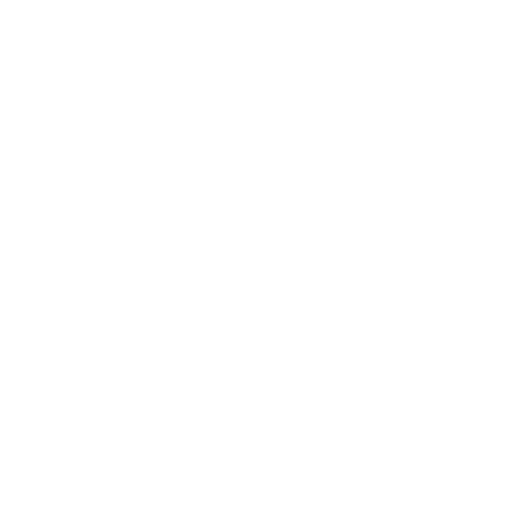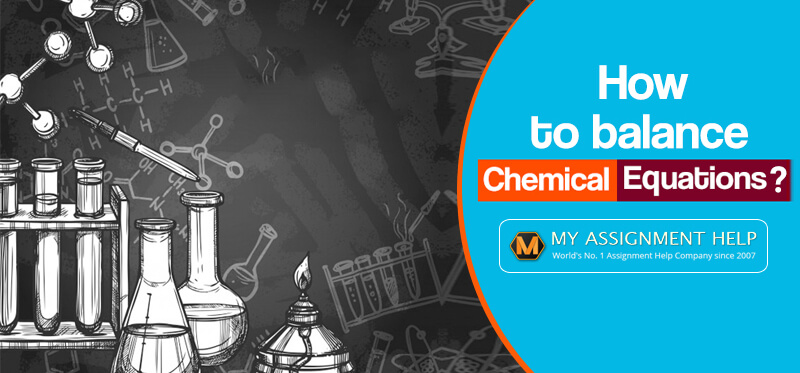\$20 Bonus + 25% OFF +
5
%
Cashback
200000+ Students

can't be Wrong!1139865 Orders4.9/5 Ratings5047 PhD Experts250 words

# Simple Guide for Balancing Chemical Equations

26 April,2019

A chemical equation is a symbolic representation of a chemical reaction. The reactant chemicals remain on the left side of the equation, and the product chemicals are on the right side of the equation. The two sides are connected with an arrow leading from the left to the right.A balanced chemical equation represents the chemical reaction using chemical formulae of reactants and products. It indicates the number of molecules or atoms of each substance.

Let’s have a look at the details of balancing chemical equations:

## Rules of Balancing Chemical Equations

Chemical reactions are like recipes. Balancing chemical reactions allows us to determine the stoichiometry calculations by understanding the ratio between reactants and products.

Let’s look at the rules of balancing chemical equations:

1. Rule 1: You can only add coefficients while balancing chemical equations. Always remember, that coefficients come in front of a molecule.

2H2O = 2 molecules of water.

2(H2) = 4 hydrogen atoms.

2(O) = 2 oxygen atoms.

2.   Rule 2:  Coefficients do not come in the middle of a molecule. They must always come in front of a molecule.

• 4NaCl
• Na4Cl

3.  Rule 3: You should never use subscripts or parenthesis other than a coefficient to balance the equation.

If you mean 2 water molecules, you should always write 2H2O, not H2O2.

Image 1:  Tips to balance chemical equations

4. Rule 4:  Do not change the numbers that are already a part of the chemical formula.

Let’s balance the equation:

H2 + O2 → H2O

We can start balancing the oxygen molecules. The only way this can be done is by putting a coefficient of 2 in front of the product H2O

H2+ O2= 2H2O

But the problem arises when Hydrogen atoms are no longer balanced.  Just balance it in this way:

2H2+O2 → 2H2O

## Quick method to solve the hydrobromic acid formula like a chemical equation balancer

This is a traditional method to solve the chemical equation of hydrobromic acid formula. Nowadays, you can balance the complex balance chemical equation with the help of a chemical equation balancer available online. Let’s look at the steps of a traditional method to balance a chemical equation:

1. Write down the given equation: Let us take a look at this equation:

HBr +Ca(OH)2 → CaBr2+ H2O

In this neutralisation reaction, the hydrobromic acid reacts with calcium hydroxide to yield calcium bromide and water.

2. Write down the number of atoms per element: Do this for each side of the equation. Look at the equation:

In the right side you have:

Hydrogen= 3, Bromine= 1, Calcium=1, Oxygen=2

In the left side you have:

Hydrogen= 2, Bromine=2, Calcium=1, Oxygen=1

3. Try to fix the bromines first: Let us fix the bromines first. It will balance the hydrogen as well. Let’s see:

The given equation is:  HBr +Ca (OH)2 → CaBr2+ H2O

Put a coefficient of 2 in front of the HBr. The aim is to have two bromines on the reactants side to balance the bromines of the product side. It leads to 2 Bromines on both sides.

4. Update the hydrogen:

The equation now stands: 2HBr + Ca (OH)2→CaBr2+ H2O

So there are 4 Hydrogen on the reactant side.  To balance the number of hydrogen atoms, let us put the coefficient 2 in front of the Hydrogen on the left side. The equation is:

2HBr+ Ca (OH)2→ CaBr2 + 2H2O

The hydrogen atoms are balanced on both sides.

5. Update the oxygen atoms: In the attempt to balance the hydrogen atoms, the oxygen atoms are balanced in both sides of the equation:

Let us check:

2HBr+ Ca (OH)2→ CaBr2 + 2H2O

There are 2 oxygen atoms in the right, as well as there are 2 oxygen atoms in the left.

Thus the equation of hydrobromic acid formula is now balanced.

## Balancing the equation of a hydrochloric acid formula and calcium carbonate chemical formula

Let us learn how to balance a hydrochloric acid chemical formula and carbonate chemical formula. Suppose the equation is,

CaCO3 + HCL→ CaCl2+ CO2+ H2O

Notice, there is one calcium atom on the left and one on the right. Therefore, this is balanced.

There is 1 carbon atom on the left and 1 carbon atom on the right, so this is balanced as well.

There is 1 hydrogen in the left but 2 in the right. This part is unbalanced. We need to balance it by using 2 molecules of HCL:

CaCO3+ 2HCL → CaCl2+CO2+ H20

Lastly, see whether chlorine is balanced. There are 2 on each side. Now you have a balanced hydrochloric acid formula and calcium carbonate chemical formula equation. You can also balance a hydrobromic acid formula in the same way.

## How to use an online chemical equation balancer to solve the calcium hydrogen carbonate and hydrogen chloride formula?

Instead of opting for the traditional method, you can also solve calcium hydrogen carbonate and hydrogen chloride formula with the help of an online chemical equation balancer. Let’s see how:

Image source: https://usama.site/5-online-chemical-equation-balancer-websites-free/

• Enter the equation CaCO3 + HCL→ CaCl2+ CO2+ H2O, and click balance.
• Always use upper case for the first character of the element name and the lower case for the second character.
• To enter an electron use {-} or e.
• To enter ion, specify charge after compound in early brackets: {+3} or {3+} or {3}.
• Substitute immutable groups to avoid ambiguity.
• Do not provide compound states like (s) or (aq) or (g).
• Reaction stoichiometry could be used to compute a balanced equation. Enter the number of moles or weight of one of the compounds to compute the rest in the balancer.

To know more or to balance a chemical equation, you can click here. Balancing a chemical equation is one of the most significant components of the Chemistry curriculum. Understand the rules, comprehend the guidelines, download some worksheets, and start practising them. Become an expert and fetch good grades this semester.

## Are you struggling with an assignment on Chemistry?

If writing a challenging Chemistry assignment is scaring you, then get in touch with the experts of MyAssignmenthelp.com right now. Are you unable to figure out how to memorise complicated formulae?

We, at MyAssignmenthelp.com, have a team of Chemistry experts. They are highly qualified and have decades of academic experience. They can get you out of your misery by providing flawless and accurate academic help.

Not only will they help you to fetch good grades but also assist you in achieving academic excellence. We have a high customer satisfaction rating of 4.9 out of 5.

Students hiring our services get to enjoy numerous benefits like:

• Unmatched papers
• Well-researched data
• Absolute confidentiality
• Safe payment gateway
• Unlimited Revisions
• Affordable price
• Lucrative discounts
• On time delivery
• 24*7 customer support availability

Do not waste your time struggling with a strenuous and challenging Chemistry assignment. Hire us and put an end to your academic woes.

## Related Post

####0 Comments